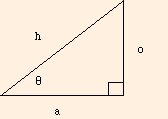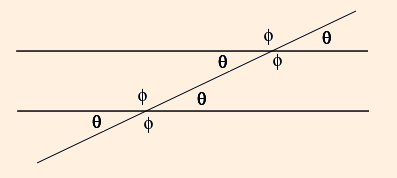### Geometry

1. The distance between two points (x1, y1, z1) and (x2, y2, z2) is given by the Pythagorean Theorem:
r = √ ((x2 - x1)2 + (y2 - y1)2 + (z2 - z1)2)
2. circumference (circle) = 2 π r
area (circle) = π r2
surface area (sphere) = 4 π r2
volume (sphere) = 4/3 π r3
surface area (cylinder) = 2 π r2 + 2 π r h
volume (cylinder) = π r2 h
3. For the right triangle:sin θ = o / h
cos θ = a / h
tan θ = o / a
4. sin (a + b) = sin a cos b + cos a sin b
cos (a + b) = cos a cos b - sin a sin b
cos2 θ + sin2 θ = 1
1 / cos2 θ = 1 + tan2 θ
5. When two parallel lines are crossed by a transverse line, the alternate interior angles are equal:(prev) (top) (next)